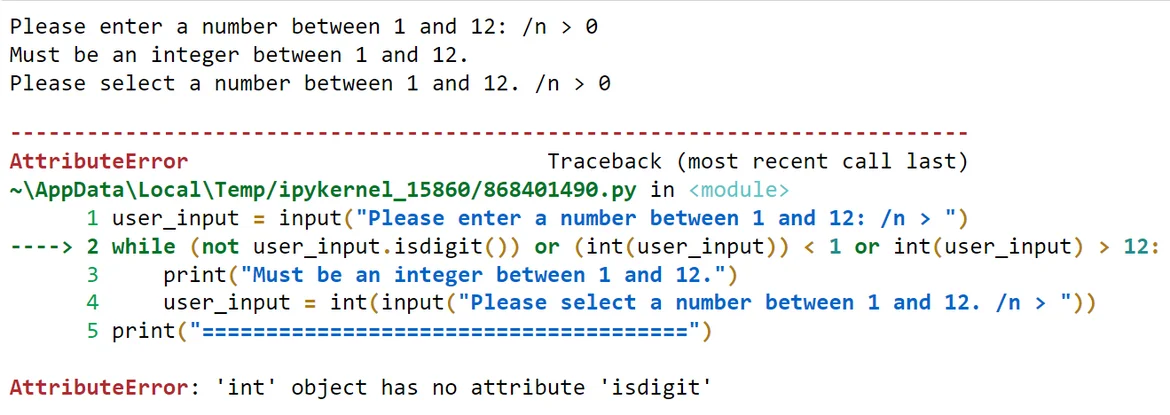08 Mar 2022

Posted on:

08 Mar 2022

0

Hi,
I'm running the following code for question 3:

user_input = input("Please enter a number between 1 and 12: /n > ")
while (not user_input.isdigit()) or (int(user_input)) < 1 or int(user_input) > 12:
print("Must be an integer between 1 and 12.")
user_input = int(input("Please select a number between 1 and 12. /n > "))
print("======================================")
print()
print(f"This is the {user_input} times table:")
print()
for i in range(1,13):
print(f"{i} x {user_input} = {i*user_input}")

But I am getting this instead:
This is the 7 times table:

1 x 7 = 7
2 x 7 = 77
3 x 7 = 777
4 x 7 = 7777
5 x 7 = 77777
6 x 7 = 777777
7 x 7 = 7777777
8 x 7 = 77777777
9 x 7 = 777777777
10 x 7 = 7777777777
11 x 7 = 77777777777
12 x 7 = 777777777777

Any ideas?

Instructor
Posted on:

08 Mar 2022

1

Hey João,

Notice that you define the variable `user_input` in two different ways. The one outside the `while`-loop is a string while the one inside the loop is an integer. This causes two problems:
1. Try entering an invalid integer twice. You should get the following error:The reason is as follows. Let's say you enter a 0 the first time (which, remember, is a string). The `while`-loop check if this string is actually a digit. It is, so it moves on to the second condition which checks if the digit is smaller than 1. It is, so you enter the loop. Now, the second time you enter a 0 (which is now directly converted to an integer), you again need to go through the conditions of the `while`-loop. First, you check whether your integer is ... an integer :) As it turns out, integers don't have the attribute `isdigit()`, so an error is thrown. The remedy is to define `user_input` as a string in both cases, namely:

``````user_input = input("Please enter a number between 1 and 12: /n > ")
while (not user_input.isdigit()) or (int(user_input)) < 1 or int(user_input) > 12:
print("Must be an integer between 1 and 12.")
user_input = input("Please select a number between 1 and 12. /n > ")
``````

2. The second trouble is the following. In the lucky occasion of entering a valid input from the first time, you won't enter the while-loop. Therefore, `user_input` will be left as a string. The command `{i*user_input}` inside the very last `print` function multiplies this string `i` times. This translates into printing out the number `i` many times. Modifying the print function as follows should solve the problem:

``````print(f"{i} x {user_input} = {i*int(user_input)}")
``````

Hope this helps! Keep up the good work!

Kind regards,
365 Hristina

Posted on:

08 Mar 2022

0

Awesome! Thanks a lot!!